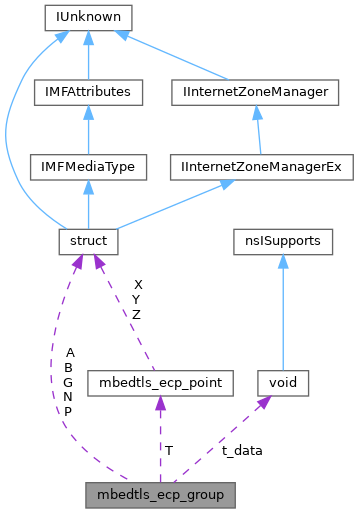ReactOS 0.4.15-dev-7108-g1cf6ce6
mbedtls_ecp_group Struct Reference

The ECP group structure. More...

`#include <ecp.h>`

Collaboration diagram for mbedtls_ecp_group:[legend]

## Public Attributes

mbedtls_ecp_group_id id

mbedtls_mpi P

mbedtls_mpi A

mbedtls_mpi B

mbedtls_ecp_point G

mbedtls_mpi N

size_t pbits

size_t nbits

unsigned int h

int(* modp )(mbedtls_mpi *)

int(* t_pre )(mbedtls_ecp_point *, void *)

int(* t_post )(mbedtls_ecp_point *, void *)

voidt_data

mbedtls_ecp_pointT

size_t T_size

## Detailed Description

The ECP group structure.

We consider two types of curve equations:

• Short Weierstrass: `y^2 = x^3 + A x + B mod P` (SEC1 + RFC-4492)
• Montgomery: `y^2 = x^3 + A x^2 + x mod P` (Curve25519, Curve448)

In both cases, the generator (`G`) for a prime-order subgroup is fixed.

For Short Weierstrass, this subgroup is the whole curve, and its cardinality is denoted by `N`. Our code requires that `N` is an odd prime as mbedtls_ecp_mul() requires an odd number, and mbedtls_ecdsa_sign() requires that it is prime for blinding purposes.

For Montgomery curves, we do not store `A`, but `(A + 2) / 4`, which is the quantity used in the formulas. Additionally, `nbits` is not the size of `N` but the required size for private keys.

If `modp` is NULL, reduction modulo `P` is done using a generic algorithm. Otherwise, `modp` must point to a function that takes an `mbedtls_mpi` in the range of `0..2^(2*pbits)-1`, and transforms it in-place to an integer which is congruent mod `P` to the given MPI, and is close enough to `pbits` in size, so that it may be efficiently brought in the 0..P-1 range by a few additions or subtractions. Therefore, it is only an approximative modular reduction. It must return 0 on success and non-zero on failure.

Note
Alternative implementations must keep the group IDs distinct. If two group structures have the same ID, then they must be identical.

Definition at line 232 of file ecp.h.

## ◆ A

 mbedtls_mpi mbedtls_ecp_group::A

For Short Weierstrass: `A` in the equation. For Montgomery curves: `(A + 2) / 4`.

Definition at line 236 of file ecp.h.

## ◆ B

 mbedtls_mpi mbedtls_ecp_group::B

For Short Weierstrass: `B` in the equation. For Montgomery curves: unused.

Definition at line 238 of file ecp.h.

## ◆ G

 mbedtls_ecp_point mbedtls_ecp_group::G

The generator of the subgroup used.

Definition at line 240 of file ecp.h.

## ◆ h

 unsigned int mbedtls_ecp_group::h

Definition at line 246 of file ecp.h.

## ◆ id

 mbedtls_ecp_group_id mbedtls_ecp_group::id

An internal group identifier.

Definition at line 234 of file ecp.h.

## ◆ modp

 int(* mbedtls_ecp_group::modp) (mbedtls_mpi *)

The function for fast pseudo-reduction mod `P` (see above).

Definition at line 247 of file ecp.h.

## ◆ N

 mbedtls_mpi mbedtls_ecp_group::N

The order of `G`.

Definition at line 241 of file ecp.h.

## ◆ nbits

 size_t mbedtls_ecp_group::nbits

For Short Weierstrass: The number of bits in `P`. For Montgomery curves: the number of bits in the private keys.

Definition at line 243 of file ecp.h.

## ◆ P

 mbedtls_mpi mbedtls_ecp_group::P

The prime modulus of the base field.

Definition at line 235 of file ecp.h.

## ◆ pbits

 size_t mbedtls_ecp_group::pbits

The number of bits in `P`.

Definition at line 242 of file ecp.h.

## ◆ T

 mbedtls_ecp_point* mbedtls_ecp_group::T

Pre-computed points for ecp_mul_comb().

Definition at line 252 of file ecp.h.

## ◆ t_data

 void* mbedtls_ecp_group::t_data

Unused.

Definition at line 251 of file ecp.h.

## ◆ t_post

 int(* mbedtls_ecp_group::t_post) (mbedtls_ecp_point *, void *)

Unused.

Definition at line 250 of file ecp.h.

## ◆ t_pre

 int(* mbedtls_ecp_group::t_pre) (mbedtls_ecp_point *, void *)

Unused.

Definition at line 249 of file ecp.h.

## ◆ T_size

 size_t mbedtls_ecp_group::T_size

The number of pre-computed points.

Definition at line 253 of file ecp.h.

The documentation for this struct was generated from the following file:
• sdk/include/reactos/libs/mbedtls/ecp.h